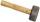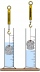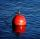# Hollow sphere

The steel hollow sphere floats on the water plunged into half its volume. Determine the outer radius of the sphere and wall thickness, if you know that the weight of the sphere is 0.5 kg and the density of steel is 7850 kg/m3

r =  6.2 cm
h =  0.1346 cm

### Step-by-step explanation:Did you find an error or inaccuracy? Feel free to write us. Thank you!Tips to related online calculators
Tip: Our volume units converter will help you with the conversion of volume units.

#### You need to know the following knowledge to solve this word math problem:

We encourage you to watch this tutorial video on this math problem:

## Related math problems and questions:

• Wall thicknessThe hollow metal ball has an outside diameter of 40 cm. Determine the wall thickness if the weight is 25 kg and the metal density is 8.45 g/cm3.
• Iron sphereIron sphere has weight 100 kg and density ρ = 7600 kg/m3. Calculate the volume, surface, and diameter of the sphere.
• Brass tubeThe outer perimeter of brass tube (ρ = 8.5 g/cm3) is 38 cm. Its mass is 5 kg, length 54 cm. What is the pipe wall thickness?
• SeawaterSeawater has a density of 1025 kg/m3, ice 920 kg/m3. 8 liters of seawater froze and created a cube. Calculate the size of the cube edge.
• Cuboid 5Calculate the mass of the cuboid with dimensions of 12 cm; 0.8 dm and 100 mm made from spruce wood (density = 550 kg/m3).
• Hollow sphereCalculate the weight of a hollow stříbrné sphere (density 10.5 g/cm3) if the vnitřní diameter is 15 cm and wall thickness is 1 mm.
• Cone from cubeFrom a wooden block, 20 cm high was the turned largest possible cone. Calculate its weight if you know that the density of wood was 850 kg/m3
• A steelA steel hammer with a weight of 0.5 kg hits the nail at a speed of 3 m/s. How deep the nail penetrates the wood if the hammer acts on the nail with an average force of 50N.
• Above water surfaceIf we remove the stone from the water, we apply a force of 120N. How much force will we have to exert if we move the stone above the water surface? The density of the stone is 5000 kg/m ^ 3.
• Eiffel TowerEiffel Tower in Paris is 300 meters high and is made of steel. Its weight is 8000 tons. How tall is the tower model made ​​of the same material if it weighs is 2.4 kg?
• SphereThe surface of the sphere is 12100 cm2, and the weight is 136 kg. What is its density?
• Brass sphereFind the weight of a brass ball with an outer radius of 12 cm, a wall thickness of 20 mm if the brass's density is 8.5 g/cm3.
• Tower modelTower height is 300 meters, weight 8000 tons. How high is the model of the tower weight 1 kg? (State the result in the centimeters). The model is made from exactly the same material as the original no numbers need to be rounded. The result is a three-digi
• Cork and swimmingIf a person weighs 80 kg, how many kilograms of cork must take a swimming belt to float on water? The density of the human body is 1050kg/m3 and cork 300kg/m3. (Instructions: Let the human body and cork on a mixture with a density of 1000kg/m3. When you m
• Aluminum wireAluminum wire of 3 mm diameter has a total weight of 1909 kg and a density of 2700 kg/m3. How long is the wire bundle?
• Float boyaA 0.5 meter spherical float is used as a location mark for a fishing boat anchor. It floats in salt water. Find the depth to which the float sinks if the material of which the float is made weighs 8 kilograms per cubic meter and salt water weighs 1027 kg/
• Floating of wood - Archimedes lawWhat will be the volume of the floating part of a wooden (balsa) block with a density of 200 kg/m3 and a volume of 0.02 m3 that floats in alcohol? (alcohol density is 789 kg/m3)